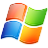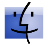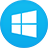# Exploration of Physical Sciences CD-ROM, Volume 2

Comprehensive software library of physical science simulations designed to provide a highly-investigative learning environment for students at a variety of levels.

By buying this product you can collect up to 105 loyalty points. Your cart will total 105 points that can be converted into a voucher of \$15.75.

\$272.16 tax excl.

### Volume discounts

QuantityDiscountYou Save
2 3% Up to \$16.33
3 5% Up to \$40.82
•Exploration of Physical Science: Simulation Library Vol. II is a vast collection of 100 computer simulations encompassing a full-range of physical science topics. Developed by Dr. Carroll and Dr. Amiri of Weber State University, the software program utilizes a conceptual approach to teach physical science principles. The simulations are categorized into three learning levels: 1) introductory, 2) intermediate, and 3) advanced; addressing the needs of introductory physical science, high school physics, and college physics courses. The multi-level learning feature gives the software package a great deal of flexibility to meet a wide range of student needs. The Simulation Library Vol. II collection significantly broadens the scope of the Volume I collection. Each simulation re-creates a real world physical event, with the student given full control over the relevant experimental variables. Experimental parameters are easily manipulated using an assortment of slider controls; physical behaviours are animated on-screen using graphics that employ rich colour and depth; and physical quantities are displayed using digital readouts, graphs, and histograms. Each simulation has a readily accessible help screen providing information on using the simulation.

The vast collection of simulations may be used in a variety of ways: (1) as an instructor lecture aid for demonstration purposes in front of the classroom, (2) for student use as a computer-based lab activity. Exploration of Physical Science simulations can be used to introduce a physical science concept, or serve nicely to reinforce and extend a lab (involving apparatus) that has already been performed. The ready-to-run simulations and highly intuitive interface allows first time users to immediately use the simulations and begin exploring with no preliminary time investment - essentially providing a ready to go lab experience.

Simulations Contained in Volume 2:

Forces and Motion - A car’s linear velocity and acceleration graphs; Racing cars: Distance, velocity, and acceleration; Galileo’s experiment: Falling and air resistance; Velocity and acceleration of a falling ball; Falling balls with air resistance; Free fall: Independence of velocity components; Throwing a banana to a falling monkey; Projectile motion: Horizontal and vertical motion; Projectile motion and acceleration; Trajectory of a ball with air resistance; Newton’s 1st law: Puck on moving ice sheet; Newton’s 2nd law: A dogsled race; Static and kinetic friction; Skidding cars and stopping distances; Air resistance with one parachute; Air resistance with two racing parachutes; Newton’s 3rd law: Two astronauts playing catch; Newton’s 3rd law: Rocket propulsion; Centre of mass of a drawn figure; Balancing people on a seesaw; Circular motion of a car on a race track; Circular motion of a sling: Tension and gravity; Angular momentum on a merry-go-round.

Momentum and Energy - Jumping from a cart: Conservation of momentum; Inelastic car crash in two-dimensions; Energy conservation of a falling ball; Energy conservation on a loop-the-loop; Energy conservation of a pendulum; Energy conservation of a mass on a spring; Bouncing balls and the coefficient of restitution; Elastic & inelastic colliding balls in one-dimension; Colliding balls in two-dimensions; Energy conservation of a bungee jumper; Effect of friction on a car rolling on inclined surfaces; Effects of friction/air resistance on skiing snowman.

Thermodynamics - The three phases of water and latent heat; The ideal gas law; Temperature, speed, and kinetic energy; The distribution of molecular speeds in a gas; Mixing in a box of gas particles; Entropy and the 2nd law of thermodynamics.

Vibrations, Waves and Sounds - Simple harmonic motion and the sine function; Simple harmonic and circular motion; Resonance of a damped, driven mass on a spring; Normal modes: Two masses connected by springs; Wave addition: Frequency, phase, and amplitude; The superposition of waves on a rope; Standing waves & harmonics: Strings/Organ Pipes; The superposition of sound waves; The Doppler effect and sonic booms; Ripple tank interference.

Electricity and Magnetism - Static electric charges on a hanging pith ball; Charging and discharging an electroscope; Electric field lines and vectors; Trajectory of a test charge in an electric field; A light bulb and battery; Electric circuits and Ohm’s law; Measurements of series and parallel circuits; The magnetic field of bar magnets; The magnetic field of a wire and solenoid; A proton in the Earth’s magnetic field; Magnetic force on a current-carrying wire; Electric dipole radiation.

Light and Optics - Fizeau’s experiment and the speed of light; The polarization of light and polarizing filters; The refraction of waves at a boundary; The refraction of light by prisms and raindrops; Additive and subtractive mixing of colours; Light rays and the formation of a real image; Ray tracing: Lenses and mirrors (5 simulations); Single-slit diffraction of light; Interference of light waves from two slits; Double-slit interference and diffraction patterns.

Relativity - The Michelson-Morley experiment; Relativity and simultaneity for a moving train; Length contraction; Time dilation; Racing trains: Newton’s vs. Einstein’s mechanics.

Modern Physics - Radioactive decay; The photoelectric effect: Measuring 5 metals; Double-slit electron interference; Three models of the atom; The atomic nucleus and Rutherford’s experiment; Measurements of the quantum atom; The structure of matter: A salt crystal; The chemical bond.

Astronomy - Retrograde motion in geocentric/heliocentric systems, Planetary motion: Kepler’s laws, The motion of a satellite orbiting Earth.

Chaos and Fractals
- Motion of a chaotic pendulum, Sierpinski triangle; Pythagorean Tree. Fluids - Measuring pressure in liquids; Mass, volume, density, and buoyancy

System Requirements: Windows: Windows XP/7/8/10. Macintosh: Mac OSX  10.6 - 10.11.